The angular impulse-momentum relation

Angular momentum of a particle: The symbol HO denotes the angular momentum and is defined as the moment of linear momentum around the point O, and given by the equation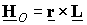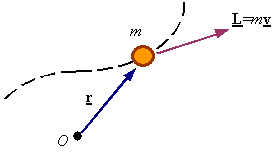Impulse of a moment from time t1 to t2: The integral of the moment over the time interval of concern is its impulse. The impulse of a moment is a vector given by the integral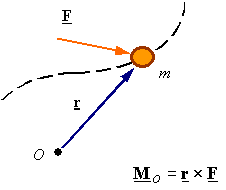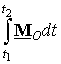Moment and rate-of-change-of angular momentum relation: Starting from Newton’s second law, calculating the moment of both sided of the equation with respect to a point O on an inertial frame results in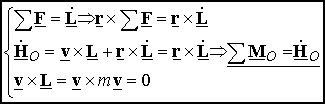This resulting relation between the moment and the rate of change of angular momentum is called the moment-rate of change of angular momentum relation (MRCAM).

The angular impulse-momentum relation: Integration of Newton’s 2nd law over the time interval from t1 to t2 results in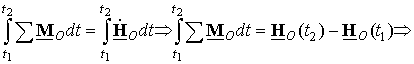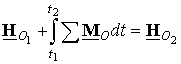Therefore, the angular momentum of a particle is changed by the impulse of the resultant moment on the particle. There will be conservation of angular momentum only if the impulse of the resultant moment is zero.